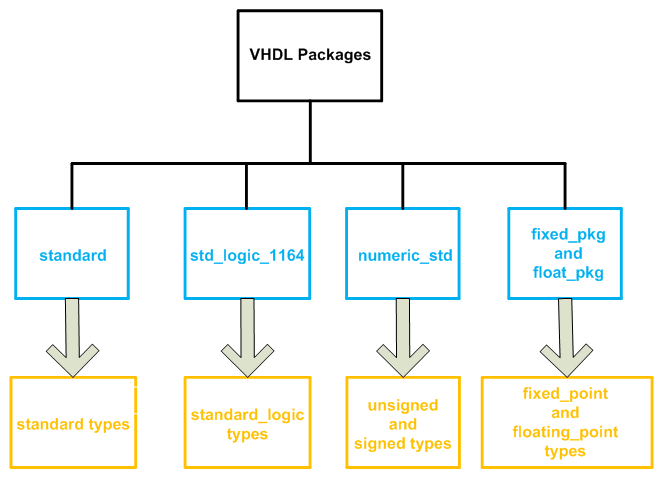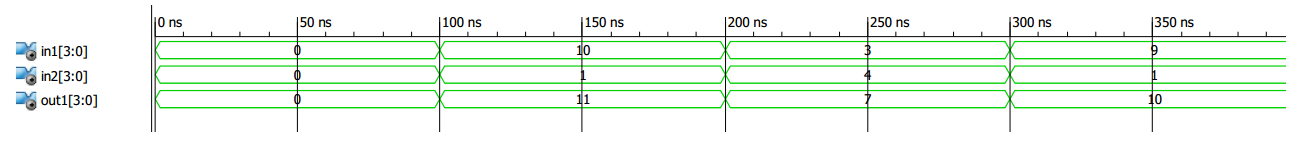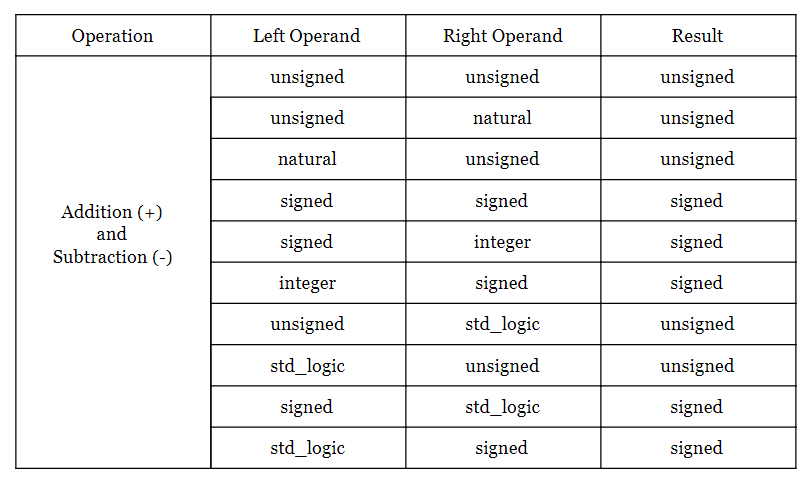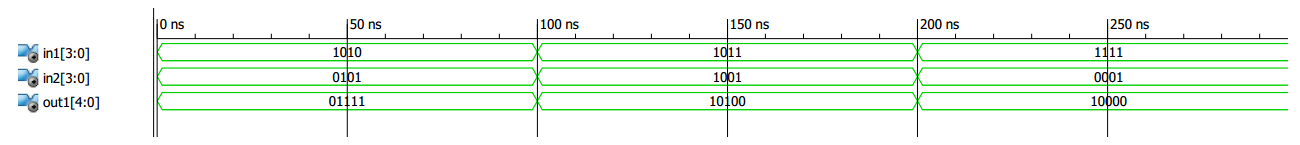Technical Article

# Review of VHDL Signed/Unsigned Data Types

February 01, 2018 by Dr. Steve Arar

In recent articles we’ve looked at some important VHDL data types: std_logic, std_logic_vector, bit, boolean, and integer. This article will review the “signed”/“unsigned” data types, which can be used when dealing with whole numbers.

### Signed/Unsigned Data Types

As shown in Figure 1, the signed/unsigned data types are defined in the “numeric_std” package. This package is included in the “ieee” library.##### Figure 1. The “signed” and “unsigned” data types are defined in the numeric_std package.

To use “signed” and “unsigned” data types, we need to include the following lines in our code:

1	library ieee;
2	use ieee.std_logic_1164.all;
3	use ieee.numeric_std.all;

Note that the “std_logic_1164” package is required because the “numeric_std” package uses the “std_logic” data type. In fact, similar to the “std_logic_vector” data type, the “signed” and “unsigned” data types are a vector of elements of type “std_logic”. However, unlike the “std_logic_vector” type, the “signed” and “unsigned” types have a numeric interpretation. Consider the following code:

1	signal slv1 : std_logic_vector(2 downto 0);
2	signal sig1 : signed(2 downto 0);
3	signal usig1 : unsigned(2 downto 0);
4	slv1 <= “101”;
5	usig1 <= “101”;
6	sig1 <= “101”;

The above code defines three signals, slv1, sig1, usig1, of type “std_logic_vector”, “signed”, and “unsigned”, respectively. Then, it assigns “101” to these three signals. As discussed in a previous article, we cannot assume a weight for the different bit positions of a “std_logic_vector”. Hence, slv1 only represents a sequence of ones and zeros, with no other interpretation. However, usig1 and sig1 have numeric interpretations: usig1 is interpreted as the unsigned binary representation of 5, and sig1 is interpreted as -3. Note that VHDL uses the two’s complement technique to represent negative values.

Since the types “signed” and “unsigned” use “std_logic” as their base type, we can assign an element of type “signed”/“unsigned” to a “std_logic” object. For example, if s1 is declared as a “std_logic” signal, the following assignment is valid:

s1 <= sig1(1);

While the “std_logic_vector”, “signed”, and “unsigned” data types are closely related, we need to be careful when assigning these data types to each other. For example, the following assignments are illegal:

usig1 <= slv1;
sig1 <= slv1;
sig1 <= usig1;

For these assignments, we have to first perform type casting and then the assignment operation. Type casting is a way to convert an object from one data type to another data type. Type casting and type conversion will be discussed in a future article.

### Arithmetic Operations with “Signed”/“Unsigned” Types

The package “numeric_std” defines arithmetic operations for the “signed” and “unsigned” data types. The following code is an example where two four-bit unsigned objects are added together.

1	library IEEE;
2	use IEEE.STD_LOGIC_1164.ALL;
3	use IEEE.NUMERIC_STD.ALL;

4	entity SignedTest1 is
5				port(in1, in2 : in unsigned(3 downto 0);
6				     out1 : out unsigned(3 downto 0));
7	end SignedTest1;

8	architecture Behavioral of SignedTest1 is

9	begin

10		out1 <= in1 + in2;

11	end Behavioral;

Figure 2 shows a simulation of this code. Note that this figure represents the decimal equivalent of the values to simplify verification of the simulation result.##### Figure 2.

There are two points that need further attention:

First, the numeric operations are generally defined such that the data path width does not change. For example, in the above code two four-bit numbers are added together and the result is assigned to another four-bit object. Consequently, we have to account for the possibility of overflow (because two four-bit numbers can produce a five-bit sum). If we resize the inputs and represent them with one extra bit, then overflow will not occur. This will be further discussed in the upcoming examples.

Second, the above example shows that we can add two “unsigned” values together, but what other options are there? For example, does VHDL allow us to mix the types and add an “unsigned” value to a “signed” one? Does it allow us to add a “signed” or “unsigned” value to the value represented by a “std_logic” signal? It turns out that the left and right operands of the addition (and subtraction) operator can be as listed in the following table. The table also shows the type of the result.As mentioned above, we can increase the length of signals by one bit if we want to prevent overflow. For example, when dealing with “unsigned” objects, we can use the following code:

1	library IEEE;
2	use IEEE.STD_LOGIC_1164.ALL;
3	use IEEE.NUMERIC_STD.ALL;

5				port(in1, in2 : in unsigned(3 downto 0);
6	           	             out1 : out unsigned(4 downto 0));

8	architecture Behavioral of Adder_NO_OV is

9	begin

10		out1 <= ('0' & in1) + ('0' & in2);

11	end Behavioral;

Since the input ports represent an unsigned value, we can append a zero after the most significant bit position of the operands without altering the represented values. This can be achieved using the concatenation operator, &, as in line 10 of the above code. In this way, we are resizing the operands to be five-bit vectors. Since the addition operation preserves the data path width, the sum will be represented using a five-bit unsigned number which is assigned to out1 in this example. Figure 3 shows an ISE simulation of this code.##### Figure 3

When working with signed numbers, we can sign-extend the operands using the concatenation operator. The following code shows how we can avoid overflow in this case.

1	library IEEE;
2	use IEEE.STD_LOGIC_1164.ALL;
3	use IEEE.NUMERIC_STD.ALL;

5				port(in1, in2 : in signed(3 downto 0);
6			             out1 : out signed(4 downto 0));

8	architecture Behavioral of Adder_NO_OV is

9	begin

10		out1 <= (in1(3) & in1) + (in2(3) & in2);

11	end Behavioral;

In this case, the ports represent “signed” numbers, so we should duplicate the sign bit rather than append zeros after the MSB.

Unlike the addition operator, the multiplication operator (*) does not preserve the data path width. The bit-width of the product is equal to the sum of the bit-widths of the two operands. For example, when multiplying an eight-bit number by a four-bit one, the result will be twelve bits long. Hence, in this case there is no overflow. However, we will generally have to truncate the result somewhere in our calculations to ensure that the data path does not become excessively wide.

### Summary

• The “signed” and “unsigned” data types are vectors of elements of type “std_logic”.
• We can assign an element of a “signed”/“unsigned” vector to a “std_logic” object.
• Numeric operations are generally defined such that the data path width does not change.
• We can prevent overflow when performing addition by increasing by one bit the length of the input signals.
• It’s important to know what data types can be used as the operands of a given operator.
• Unlike the addition operator, the multiplication operator (*) does not preserve the data path width. The bit-width of the product is equal to the sum of the bit-widths of the two operands.